# SBA 458 Nemesis® – High Repeatability and Reproducibility by Measurements on BiTeSe

#### Introduction

This application note covers the analysis of BiTeSe material using the SBA 458 Nemesis® (figure 1). The SBA 458 allows for measurement of both the Seebeck coefficient and the Conductivité Électrique (SBA)Electrical conductivity is a physical property indicating a material's ability to allow the transport of an electric charge.electrical conductivity of BiTeSe with high reproducibility and measuring accuracy. This is demonstrated by means of numerous measurement examples in the following sections. Additionally, helpful recommendations for adjusting the measurement parameters are given.

#### Design of the SBA 458 Nemesis®

With the SBA 458 Nemesis®, the Seebeck coefficient and electrical conductivity can be measured simultaneously. Figure 2 depicts the schematic design of the SBA 458. The sample lies horizontally on the sample support. On the left and right are heaters in the ceramic sample support, with which a temperature gradient can be generated in both sample directions. The thermocouples are positioned under the lower surface of the sample. These measure the thermoelectric voltage generated by the temperature gradient. Based on this, the Seebeck coefficient can be calculated. Next to the thermocouples below the lower sample surface are electrical contact points which are used to inject current into the sample. The resulting voltage is measured by means of the thermocouple legs. Using this information along with the correction functions for sample geometry, the electrical conductivity can be calculated.

#### Key Features of the SBA 458 Nemesis®

The SBA 458 Nemesis® has some key features which will be briefly discussed in the following. For a detailed description of the individual terms, our sales representative will be happy to assist you.

Plug-and-measure

A variety of sample geometries can be measured with the SBA 458. These include round samples, rod samples, thin layers, coatings and typical LFA samples. Changing out samples is a quick and uncomplicated procedure. Cumbersome interval measurements or other complicated work steps are eliminated.

Robust System

The sheathing on the thermocouples (type K, inconel coating prevents reaction or contamination of the sample and thermocouple. In addition, the thermocouples and electrical contact points are fixed. This eliminates any possibility of position shifts for the thermocouples and resulting disturbances to the measurements. This also allows for thermocouples and electrical contact points to be changed out easily.

Quality Check

It is possible to carry out a quality check for both measurement of the electrical conductivity and determination of the Seebeck coefficient. Prior to each measurement and each temperature step, it can thus be determined wether the measured value is correct or weather, for example, contact problems between the sample and thermocouples or some other problem may have falsified the measurement. This allows for high measurement accuracy to be achieved.

#### Properties and Applications Fields of BiSeTe

Bismuth telluride belongs to the telluride group. It is a gray powder also known as bimuth(III)telluride. It is categorized as a semiconductor and can be alloyed with antimony or selenium. In the present case, BiSeTe samples with the chemical composition Bi2Se0,25Te2,75 were employed. These samples have a density of 7.8 g/cm³ (rod samples) and 7.82 g/cm³ (round samples), respectively, and are type N thermoelectrics.

Figure 3 depicts the Figure of Merit of the three materials BiTe, PbTe and SiGe. It can be seen that BiTe exhibits its maximum mainly in the lower temperature range; therefore, this is where it is applied. BiSeTe is used, for example, for the cooling function of mobile coolers.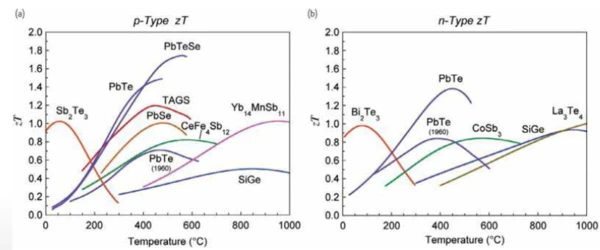3) Approximate Figure of Merit (ZT) for various p-type and n-type thermoelectric materials Source: G. Jeffrey Snyder, California Institute of Technology http://thermoelectrics.caltech.edu. Reproduced with permission.

#### Measurements on BiSeTe with the SBA 458

Repeatability

Both the Seebeck coefficient and electrical conductivity of BeSeTe can be measured with high repeatability by means of the SBA 458. To determine the repeatability, a sample is repeatedly measured under the same conditions, i.e., with the same measurement parameters. After each measurement, the sample is taken out and newly inserted. As an example for the BiSeTe sample, figures 4 and 5 show seven measurements for both the Seebeck coefficient and the electrical conductivity for the BiSeTe sample with dimensions of 3 x 1 x 15 (w x h x l). Repeatability was here determined to be at ± 2% with regard to measurement of the Seebeck coefficient, and at ± 1.5% with regard to measurement of the electrical conductivity. These values can be confirmed by measuring other BiSeTe samples (i.e., of other geometries) as well as by measuring on other SBA 458 instruments.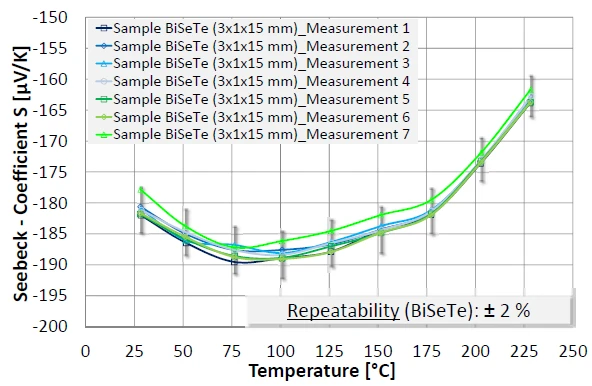4) Multiple measurement of the Seebeck coefficient of a BiSeTe sample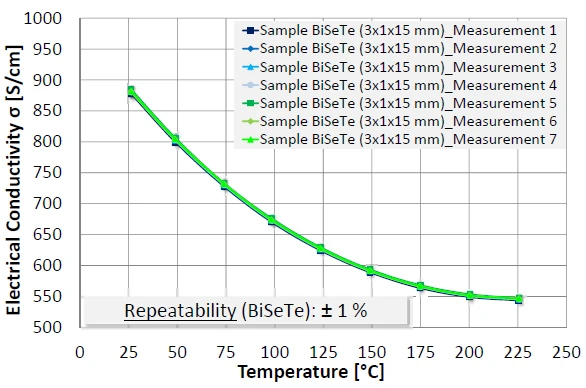5) Multiple measurement of the electrical conductivity of a BiSeTe sample

#### Reproducibility

With the SBA 458, it is possible to measure BiSeTe samples with high reproducibility as pertains to the Seebeck coefficent and electrical conductivity. This high reproducibility applies irrespective of differing sample geometries, a large amount of samples, or the implementation of measurements with different SBA 458 instruments.

As an example of this, two BiSeTe samples with different geometries were measured. A rod sample with dimensions of 3 x 1 x 15 mm (w x h x l) and a rod sample with a diameter of 12.5 x 1 mm were available for this test. Both samples were measured three times each and the values of the Seebeck coefficient and the electrical value were compared. This yielded a reproducibility of ± 2% for measurement of both the Seebeck coefficent and of electrical conductivity.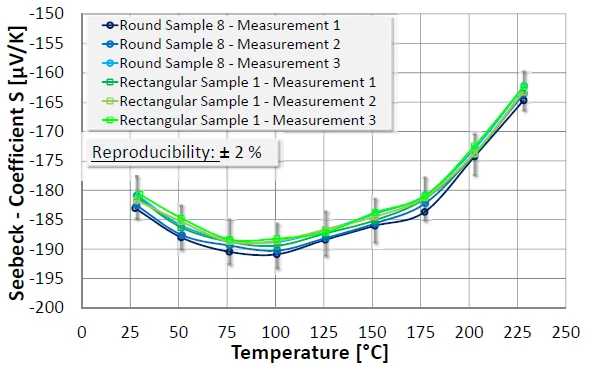6) Three measurements each of the Seebeck coefficient of a round and rod sample of the BiSeTe material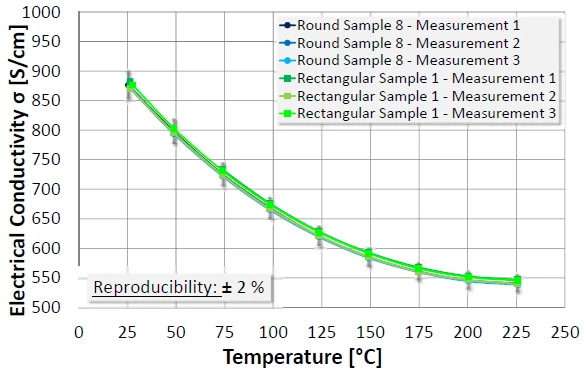7) Three measurements each of the electrical conductivity of a round and rod sample of the BiSeTe material

#### Recommendation of Suitable Measurement Parameters

All SBA 458 measurements shown here were carried out under nitrogen with a gas flow of 50 ml/min. In addition, a maximum current injection of 0.01 A and a heater voltage of 8 V were chosen as measurement parameters. These parameters have proven themselves suitable for the sample geometries employed (rod sample 3 x 1 x 15 mm, round sample with a diameter of 12.5 x 1 mm).

Since the measurement parameters are to be adjusted in part as a function of the sample material and geometry, some tips and recommendations for selecting suitable measurement parameters will be given in the following. This includes the voltage of the heater with which a temperature gradient is generated in both sample directions. Additionally, a suitable value for the maximum current injection into the sample should be selected to serve for measurement of the electrical conductivity. Furthermore, it will be shown which gases are suitable for measurement of the BiSeTe samples.

Heater Voltage

For correct measurement of the Seebeck coefficient, care should be taken when selecting the heater voltage that a sufficient temperature gradient (recommendation: ± Delta T of minimum 3 K) is generated. This can vary depending on the sample geometry. Further criteria no not need to be taken into consideration.

Current Injection

As with selecting the heater voltage, the sample geometry must be taken into consideration when determining the maximum current injection. When selecting a suitable value for the current injection, two issues must thus be kept in mind: On the one hand, the current injection should not be high enough to heat the sample, but on the other hand, the selected current must be high enough to allow for measurement of the voltage. This can be checked by a glance at the diagram with the individual values in the software. There, each of the three positive and negative current values (each 1/3, 2/3 and 3/3 of Imax) can be seen along with the respectively measured voltages. If a linear relationship can be recognized between the injected current, I, and the measured voltage, U, then a meaningful a meaningful electrical conductivity value can be measured.

Gases

For measurement of a BiSeTe sample, any of the gases allowed in the specifications for the SBA 458 is suitable.

#### Summary

For BiSeTe, the Seebeck coefficient can be measured with a repeatability of ± 2% and the Conductivité ThermiqueThermal conductivity (λ with the unit W/(m•K)) describes the transport of energy – in the form of heat – through a body of mass as the result of a temperature gradient (see fig. 1). According to the second law of thermodynamics, heat always flows in the direction of the lower temperature.thermal conductivity with a repeatability of ± 1.5% by means of the SBA 458.

Measurement of the Seebeck coefficient as well as the thermal conductivity of BiSeTe can be realized with a reproducibility of ± 2% each. This high reproducibility applies irrespective of differing sample geometries, a large amount of samples, or the implementation of measurements with different SBA 458 instruments.